﻿ Hydrostatic pressureExamples | Product | Murata Software Co., Ltd.Example57Hydrostatic pressure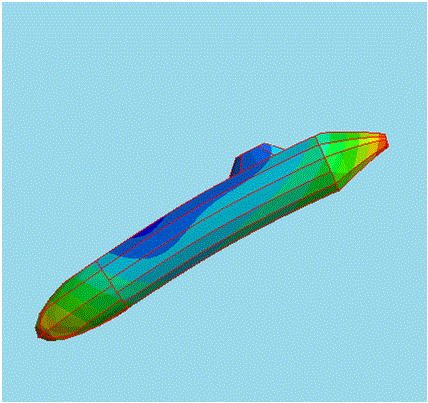General

• Deformation by the hydrostatic pressure is simulated.

• The deformation, the displacement and the mechanical stress are solved.

• Unless specified in the list below, the default conditions will be applied.

Analysis Space

 Item Settings Analysis Space 3D Model unit mm

Analysis Conditions

 Item Settings Solvers Mechanical Stress Analysis [Galileo] Analysis Type Static analysis Options N/A

As the buoyant force causes acceleration in +Z direction, setting on the High-level setting tab is done as follows.

 Tab Setting Item Setting High-level setting Setting of mechanical stress analysis and piezoelectric analysis Perform static analysis in the acceleration environment with inertial force being taken into account. is selected

Graphical Objects

A model is like a submarine going down to the sea bottom.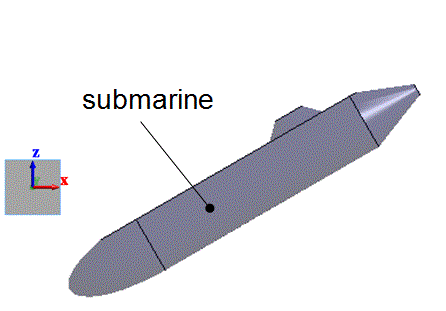Body Attributes and Materials

 Body Number/Type Body Attribute Name Material Name 0/Solid submarine 007_Fe *

* Available from the Material DB

Boundary Conditions

The hydrostatic pressure on the whole surface of the model is proportional to the depth. The pressure P is obtained in the expression below.

Pgh

where ρ is density [kg/m3], g is gravitational acceleration[m/s2], and h is depth[m]. In the case of water,

P=9800h[Pa]

• In this model, the boundary condition is only pressure. The displacement is not constrained.

Generally, the simulation cannot be performed without constraining the displacement. Femtet is, however, capable of doing so without constrained displacement.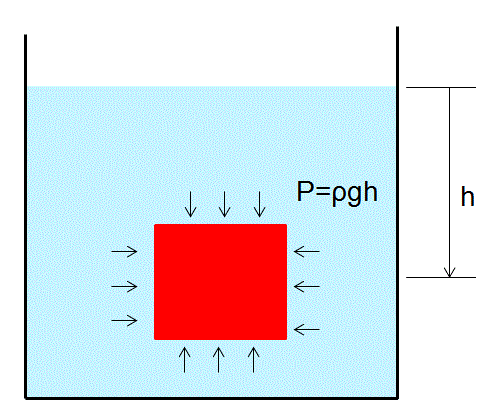For the Pressure, set “Distribution Data” of the “Edit Boundary Condition”.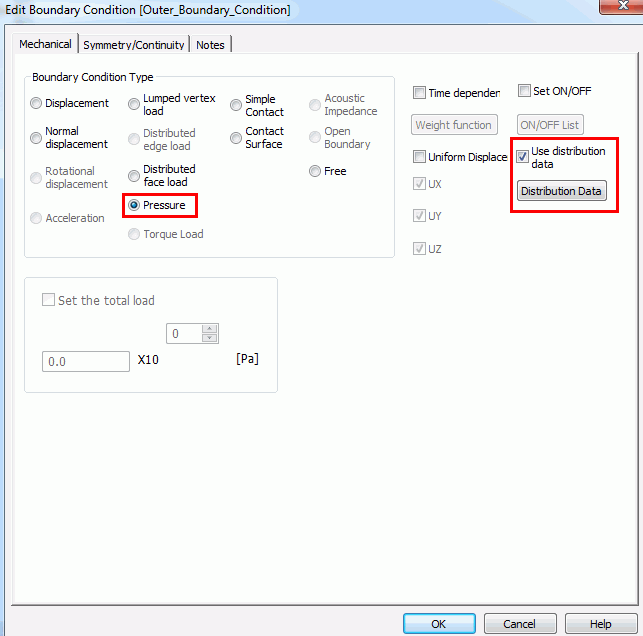In the [Edit Distribution Data] dialog box, select 1D and Z direction in the Local Coordinates. In the [Coordinates-Pressure] Table,

specify the pressures at two points in the area where the whole body is included. Linear interpolation is applied between the points.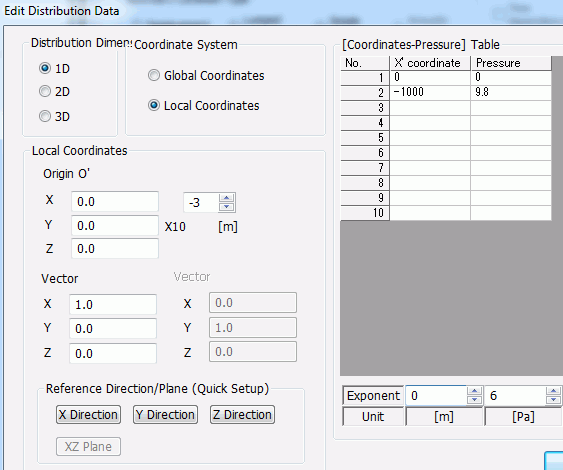After meshing, select “Distributed pressure” in the Mesh display to show the pressure distribution of the surface.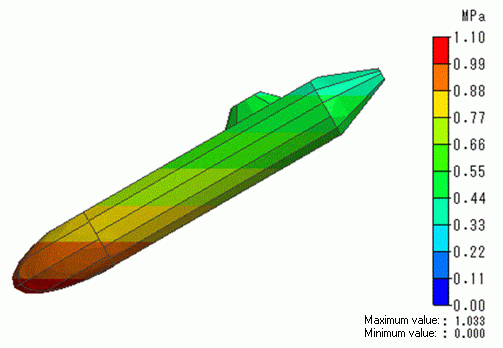Results

The displacement diagram is shown below. The contour diagram shows the magnitude of displacement.

The whole body shrinks under the pressure. The deformation is irregular due to its asymmetric shapes of upper and bottom sides of the model.

If the model is symmetric, the shrinkage will be observed uniformly over the whole body.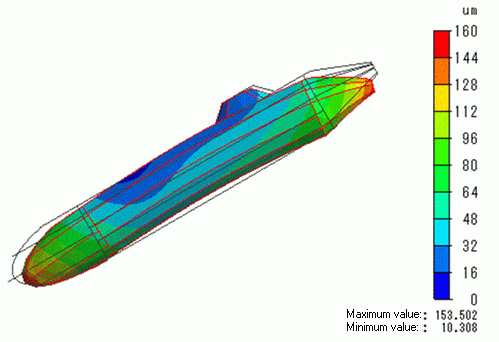﻿